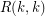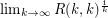Importance: Outstanding ✭✭✭✭
 Author(s): Erdos, Paul
 Subject: Combinatorics » Ramsey Theory
 Keywords: Ramsey number
 Prize: $100 /$250 (Erdos - Graham)
 Posted by: mdevos on: June 4th, 2007

Letdenote thediagonal Ramsey number.

Conjectureexists.
Problem   Determine the limit in the above conjecture (assuming it exists).

Erdos offered $100 for a solution to the highlighted conjecture and$250 for a solution to the associated problem (these prizes are now provided by Graham).

Classic results of Erdos [E] and Erdos-Szekeres [ESz] give bounds onwhich show that ifexists, then it is in the interval. Although these arguments are quite basic, little progress has been made in improving these bounds. The best known lower bound onis due to Spencer [S] and the best known upper bound is due to Thomason [T]. They are as follows:Gowers [G] has suggested that resolving these problems might require a rough structure theorem.

## Bibliography

[E] P. Erdos, Some remarks on the theory of graphs, Bull. Amer. Math. Soc. 53 (1947), 292–294. MathSciNet

[ESz] P. Erdos and G. Szekeres, A combinatorial problem in geometry, Compositio Math. 2 (1935), 463–470.

[G] W. T. Gowers, Rough structure and classification, GAFA 2000 (Tel Aviv, 1999). Geom. Funct. Anal. 2000, Special Volume, Part I, 79--117. MathSciNet

[S] J. Spencer, Ramsey’s theorem—a new lower bound, J. Comb. Theory Ser. A 18 (1975), 108–115. MathSciNet

[T] A. Thomason, An upper bound for some Ramsey numbers, J. Graph Theory 12 (1988), 509–517. MathSciNet

* indicates original appearance(s) of problem.